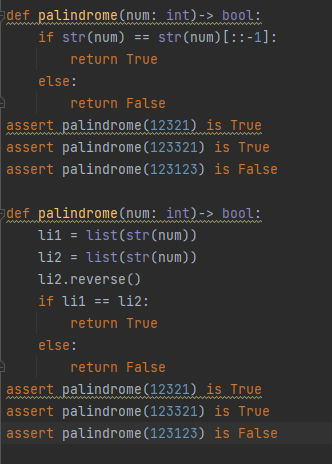# 【每日一题20220401】回文数字 #1‍ 回文是指一段数字或者文本，正着读和倒着读都是相同的，例如2002，110011都是回文数字。给定一个数字num，请编写一个函数，判断它是不是一个回文数字。

【示例】

``````def solution(num: int)-> bool:

assert solution(1221) is True
assert solution(123322) is False
assert solution(2022) is False
``````
``````def solution(num: int)-> bool:
if num == int(str(num)[::-1]):
return True
else:
return False

assert solution(1221) is True
assert solution(123322) is False
assert solution(2022) is False
assert solution(12321) is True
``````
``````def solution(num: int) -> bool:
return str(num) == str(num)[::-1]
``````
``````    if str(num)==str(num)[::-1]:
return True
else:
return False

assert solution(1221) is True
assert solution(123322) is False
assert solution(2022) is False
``````

#通过字符串倒序求解
def solution(num: int)-> bool:
num_r = int(str(num)[::-1])
return num == num_r

#通过列表倒序求解
def solution(num: int)-> bool:
lst =[i for i in str(num)][::-1]
num_r = int(’’.join(lst))
return num == num_r

``````def solution(num: int)-> bool:
return str(num)[::-1]==str(num)

``````

def solution(num):
result = True if str(num) == str(num)[::-1] else False
return result

assert solution(1221) is True
assert solution(123322) is False
assert solution(2022) is False
assert solution(“ahha”) is True
assert solution(“ahsdsha”) is False

#list方式
def solution(num: int)-> bool:
num_list = [str(x) for x in str(num)]
num_list.reverse()
revnum = int("".join(num_list))

``````if num == revnum:
return True
else:
return False
``````

assert solution(1221) is True
assert solution(123322) is False
assert solution(2022) is False

``````
def solution(num: int)-> bool:
a=int(str(num)[::-1])
return True if a ==num else False
``````
``````def palindrome(num):
return str(num)[::-1] == str(num) if isinstance(num, int) and num>=0  else 'Not valid'
``````
``````    def solution(num: int) -> bool:
str_num = str(num)
for i in range(len(str_num)//2):
if str_num[i] != str_num[-i-1]:
return False
return  True
``````
``````def solution(num: int) -> bool:
nums_str = str(num)
if nums_str == ''.join(reversed(nums_str)):
return True
else:
return False

assert solution(1221) is True
assert solution(123322) is False
assert solution(2022) is False
````````````def solution(num: int) -> bool:
if num == int(str(num)[::-1]):
return True
else:
return False

``````